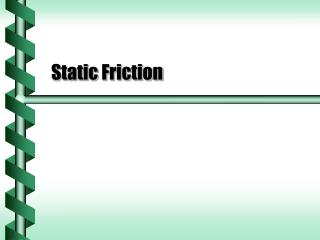# Static Friction - PowerPoint PPT PresentationDownload PresentationStatic Friction

Static FrictionDownload Presentation## Static Friction

- - - - - - - - - - - - - - - - - - - - - - - - - - - E N D - - - - - - - - - - - - - - - - - - - - - - - - - - -
##### Presentation Transcript

1. Static Friction

2. Holding in Place • Objects on an incline will often stay put. • There must be a force that holds the object in place. • Static friction is from the contact of resting objects. • Force holds up to a certain point • Force is based on the type of contact (rough, smooth) • Maximum force is proportional to the pressing force of the object (normal force)

3. Inequality • The approximate formula for static friction is: ms is the coefficient of static friction • This is an inequality. • The force of static friction is generally less than the coefficient times the normal force

4. m q Starting Motion • If Ffr < msFN = msmg cosq, then the block will hold. • At equality the block just begins to move.

5. Use a pulley and vary the weight to see when movement begins. Forces balance on mass 1 Tension is equal on the rope Forces balance on mass 2 m1 m2 Measuring Friction: Pulley FT Frope FT Frope

6. The pulley determines the coefficient of friction through the ratio of masses. m1 m2 Coefficient of Friction: Pulley

7. Use an incline and vary the angle to see when movement begins. One component of gravity balances the normal force The other component of gravity balances friction m q Measuring Friction: Incline

8. The incline determines the coefficient of friction through the tangent of the angle. m q Coefficient of Friction: Incline

9. Normal Force and Friction • Static friction depends on both the normal force and on the coefficient of friction. • To reduce friction requires reducing one of those factors. • Reduce normal force by lightening the load • Reduce normal force by adding additional upward force • Add a lubricant to reduce the coefficient of friction next